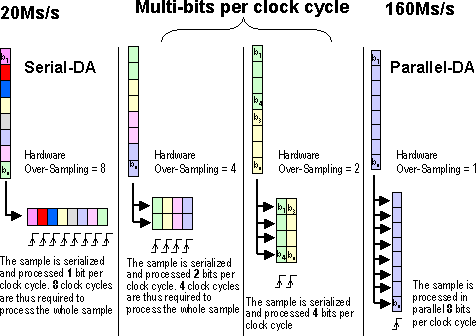# AR# 15686

|

## Description

General Description:

What does "Hardware Over Sampling" mean in regards to FIR and Multiplier blocks? Does this equate to the "Clocks per Output" parameter in the equivalent CORE Generator GUIs? What are the effects of this parameter?

## Solution

The terms "Hardware Over Sampling" and "Clocks per Output" describe the same principle in System Generator and CORE Generator. This parameter affects the following:

- Number of clock cycles needed to perform a function

- Size of the implemented design

Fundamentally, the value is a function of the input sample precision to the block and the number of bits of the input sample that are processed in one clock cycle, which directly correlates to the sample frequency of the input data.

Example 1: Sequential Structure

A 15 MHz single-rate non-symmetric 100 tap DA FIR Filter with 8-bit input data should have a "Clocks per Output" value of 8 in CORE Generator. (This value is the same as for "Hardware Over Sampling" in SysGen.) This creates a filter that requires a clock driving the logic at 120 MHz so as to achieve the 12.5 MHz sampling frequency requirement. The design will be a fully serial structure, processing 1 bit per clock cycle in the Distributed Arithmetic (DA) engine; consequently, 8 clock cycles are needed to compute the computation. The filter size will be small.

Example 2: Parallel Structure

A 100 MHz single-rate non-symmetric 100 tap DA FIR Filter with 8-bit input data should have a "Clocks per Output" value of 1 in CORE Generator. (This value is the same as for "Hardware Over Sampling" in SysGen.) This creates a filter that requires a clock driving the logic at 100 MHz so as to achieve the 100 MHz sampling frequency requirement. The design will be a fully parallel structure, processing all 8 bits of input data per clock cycle (actually eight 1-bit DA engines all working in parallel). Consequently, 1 clock cycle is needed for the computation. The size of the parallel structure will be far larger than that of the serial implementation, but far greater performance will be achieved.

Example 3: Semi-Parallel Structure

A 70 MHz Single Rate non-symmetric 100 tap DA FIR Filter with 8-bit input data should have a "Clocks per Output" value of 2 in CORE Generator. (This value is the same as for "Hardware Over Sampling" in SysGen.) This creates a filter that requires a clock driving the logic at 140 MHz so as to achieve the 70 MHz sampling frequency requirement. The design will create a 4-bit DA engine (actually four 1-bit DA engines working in parallel) that requires two clock cycles to process the complete 8-bit sample. The size of this design will be larger than that of a purely serial implementation, but smaller than that of the fully parallel implementation.

The following diagram represents this concept:Illustration of the "Hardware Over Sampling" parameter effect

The "Hardware Over Sampling" parameter in System Generator and its equivalent "Clocks per Output" parameter in CORE Generator can be used to directly control the size and performance of DA FIR filters. The same concepts apply to the slice-based multiplier structure as well. An advantage to using a DA FIR implementation is that the performance of the filter is not dependent on the number of taps (like a MAC engine), but on the width of the input data, which is generally smaller than the number of taps in a filter.

Important Exceptions to These Rules

Symmetric Coefficients

If the coefficients have a symmetric impulse response, as is often the case in FIR filter design due to the linear phase effects of symmetric coefficients, the data input width to the DA engine is increased by one. This is caused by the optimization in the filter structure that can be achieved from symmetric coefficients, which nearly halves the number of multiplications required to achieve the result. To achieve this optimization, a pre-adder is required before the DA engine -- this increases the input data width by 1-bit.

For example:

A 44 MHz single-rate symmetric 100 tap DA FIR Filter with 8-bit input data should have a "Clocks per Output" value of 3 in CORE Generator. (This value is the same as for "Hardware Over Sampling" in SysGen.) This creates a filter that requires a clock driving the logic at 132 MHz so as to achieve the 44 MHz sampling frequency requirement. The design will create a 3-bit DA engine (actually three 1-bit DA engines working in parallel) that takes 3 clock cycles to process the complete 9-bit (8+1 = 9) sample.

NOTE: See the DA FIR v8.0 data sheet for more details.

Polyphase Implementations

In polyphase implementations of the DA FIR that implement decimation or interpolation functions, the filters always operate at a frequency equal to that of the filter's slower operating frequency. Consequently, fully parallel filter structures should never be used in polyphase implementations.

For example:

A 4:1 Polyphase decimator with a 100 MHz input sample rate non-symmetric 100 tap DA FIR Filter with 10-bit input data should have a "Clocks per Output" value of 5 in CORE Generator. (This value is the same as for "Hardware Over Sampling" in SysGen.) This is necessary because the individual phase filters operate at the slower output frequency of 25 MHz. Consequently, a more serial implementation can be used to implement these filters. The 10-bit samples can be operated on by five 2-bit DA engines in the time required. The filter requires a clock driving the logic at 125 MHz so as to achieve the 25 MHz sampling frequency requirement of the phase filters.

NOTE: See the DA FIR v8.0 or Multiplier Generator data sheets for more information.

AR# 15686
Date 12/15/2012
Status Active
Type General Article
People Also Viewed